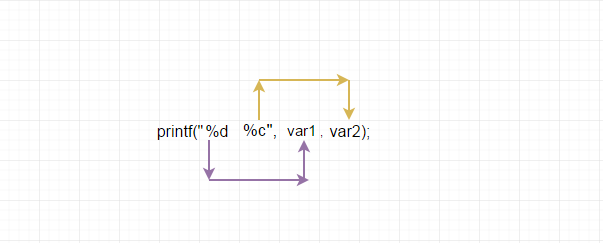# Printing variables

Using printf function, we can print the value of a variable.

In order to print the variable value, we must instruct the printf function the following details,

1.specify the format of variable.

2.variable name which we want to print.

# Basic format specifiers in C

Format Specifier

Data Type

description

Syntax

%d

int

To print the integer value

```printf("%d",<int_variable>);
```

%f

float

To print the floating number

```printf("%f",<float_variable>);
```

%lf

double

To print the double precision floating number
or long float

```printf("%lf",<double_variable>);
```

%c

char

To print the character value

```printf("%c",<char_variable>);
```

Example

```#include<stdio.h>

int main()
{
int  i = 10;
char c = 'a';

printf("Value of i = %d\n",i);
printf("Value of c = %c\n",c);

return 0;
}
```

Some other format specifiers also available in C. We will discuss about it, when it needed.

## Multiple format specifiers in same printf

we can use many format specifiers in the same printf function.

#### How it works?

if printf encounter any format specifier first time, it will take the first variable after the comma "," and print it. Next time, It will take the second variable after the comma and print it and so on.

Example

#### Pictorial ExplanationExample

```#include<stdio.h>

int main()
{
int age = 10;
char initial = 'A';
float weight = 25.5;

printf("age is \t %d\n",age);
printf("initial is \t %c\n",initial);
printf("weight is \t %f\n",weight);

//or we can print everything on the same line

printf("age=%d \t initial=%c \t weight=%f\n",age,initial,weight);

return 0;
}
```

## Useful Resources

https://en.wikipedia.org/wiki/Printf_format_string